Next: Model of Ptolemy Up: Geometric Planetary Orbit Models Previous: Model of Kepler

# Model of Hipparchus

Hipparchus' geometric model of the apparent orbit of the sun around the earth can also be used to describe a heliocentric planetary orbit. The model is illustrated in Fig. 18. The orbit of the planet corresponds to the circle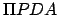(only half of which is shown), whereis the perihelion point,the planet's instantaneous position, andthe aphelion point. The diameter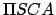is the effective major axis of the orbit (to be more exact, it is the line of apsides), where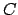is the geometric center of circle, and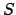the fixed position of the sun. The radiusof circleis the effective major radius,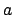, of the orbit. The distance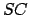is equal to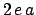, where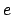is the orbit's effective eccentricity. The angle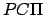is identified with the mean anomaly,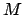, and increases linearly in time. In other words, as seen from, the planetmoves uniformly around circlein a counterclockwise direction. Finally,is the radial distance,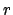, of the planet from the sun, and angleis the planet's true anomaly,.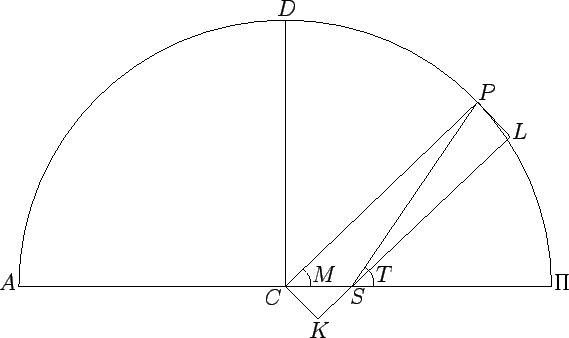Let us draw the straight-line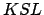parallel to, and passing through point, and then complete the rectangle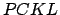. Simple geometry reveals that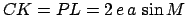,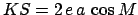, and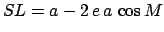. Moreover,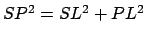, which implies that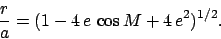(83)

Now,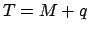, where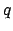is angle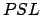. However,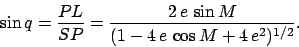(84)

Finally, expanding the previous two equations to second-order in the small parameter, we obtain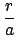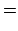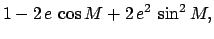(85)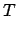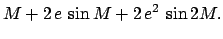(86)

It can be seen, by comparison with Eqs. (81) and (82), that the relative radial distance,, in the Hipparchian model deviates from that in the (correct) Keplerian model to first-order in(in fact, the variation ofis greater by a factor ofin the former model), whereas the true anomaly,, only deviates to second-order in. We conclude that Hipparchus' geometric model of a heliocentric planetary orbit does a reasonably good job at predicting the angular position of the planet, relative to the sun, but significantly exaggerates (by a factor of) the variation in the radial distance between the two during the course of a complete orbital rotation.Next: Model of Ptolemy Up: Geometric Planetary Orbit Models Previous: Model of Kepler
Richard Fitzpatrick 2010-07-21